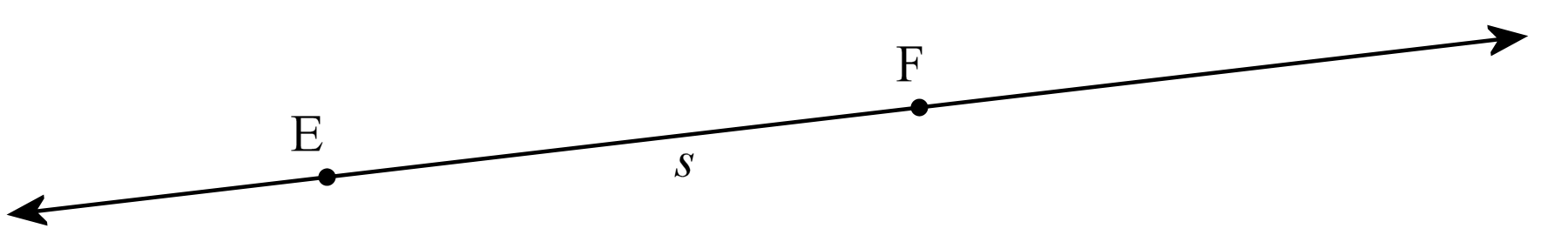# Line, Angle, Circle

Lines, angles, and circles are the basic part of geometry. Their basic concept and definitions are given here.

## Line

A line has no beginning point or ending point. You could imagine it continuing indefinitely in both directions. We can illustrate that by little arrows on both ends.We can name a line using two points on it. This is line EF. Or, we can name a line using a lowercase letter: this is line s.

## Angle:

Two lines that make an angle are called rays.  A ray starts out at a point and continues off to infinity. Angles are measured in degrees, each degree is 1/360 or a round trip (circle).

1. Acute Angle: Angle greater than 0 degree and less than 90 degrees is called acute angle.

2. Right Angle: Angle that is exactly 90 degrees is called right angle. Right angles are usually represented by a box in the corner of the angle.

3. Obtuse Angle: Angle that is greater 90 degrees and less than 180 degrees are called obtuse angle.

4. Straight Angle: Angle that is exactly 180 degrees is called a straight angle.

5. Reflective Angle: An angle that is greater than 180 degrees and less than 360 degrees is called reflective angles.

6. Full Rotation: It is exactly 360 degree.

7. Complementary Angle: Angles that add up to 90 degrees are called complementary angles. If x+y=90 degrees then ‘x’ and ‘y’ are complementary angles.

8. Supplementary Angle: Angles that add up to 180 degrees are called supplementary angles. If x+y=180 degrees then ‘x’ and ‘y’ are supplementary angles.

9. Vertical Angle: Angles that are opposite to one another are called vertical angles and are equal.## Circles

A line from the center of a circle to the circumference of the circle is called a circle.

Radius: A line from the center (also know the midpoint) of a circle to the circumference of the circle is called a radius.

Chord: A line connecting any two points on a circle is called a chord. The longest chord in a circle is the diameter.

Diameter: The longest chord in a circle is the diameter. or a line going from one side of the circle to other and through the midpoint is called the diameter. A diameter is equal to two radii.

Circumference: It is the distance once around the circle. The circumference, which is the perimeter of the circle is 2π r or π d (π multiplied by the diameter, remember that the diameter is the same as two radii.

Circumference = π × Diameter               or

Circumference = 2 × π × RadiusThe area of a circle is π given by

A = π r2

nc